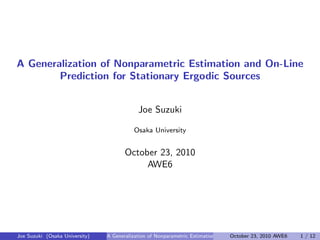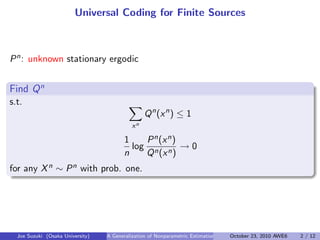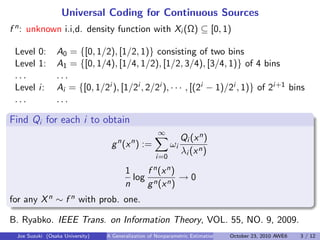Successfully reported this slideshow.

# A Generalization of Nonparametric Estimation and On-Line Prediction for Stationary Ergodic Sources

0

Share×
1 of 12
1 of 12

# A Generalization of Nonparametric Estimation and On-Line Prediction for Stationary Ergodic Sources

0

Share

Joe Suzuki, Osaka University
October 23, 2010, AWE6

Joe Suzuki, Osaka University
October 23, 2010, AWE6

## More Related Content

### Related Books

Free with a 14 day trial from Scribd

See all

### Related Audiobooks

Free with a 14 day trial from Scribd

See all

### A Generalization of Nonparametric Estimation and On-Line Prediction for Stationary Ergodic Sources

1. 1. A Generalization of Nonparametric Estimation and On-Line Prediction for Stationary Ergodic Sources Joe Suzuki Osaka University October 23, 2010 AWE6 Joe Suzuki (Osaka University) A Generalization of Nonparametric Estimation and On-Line Prediction for Stationary ErgodOctober 23, 2010 AWE6 1 / 12
2. 2. Universal Coding for Finite Sources Pn: unknown stationary ergodic   Find Qn . s.t. ∑ xn Qn (xn ) ≤ 1 1 n log Pn(xn) Qn(xn) → 0 for any Xn ∼ Pn with prob. one. Joe Suzuki (Osaka University) A Generalization of Nonparametric Estimation and On-Line Prediction for Stationary ErgodOctober 23, 2010 AWE6 2 / 12
3. 3. Universal Coding for Continuous Sources f n: unknown i.i,d. density function with Xi (Ω) ⊆ [0, 1)   Level 0: A0 = {[0, 1/2), [1/2, 1)} consisting of two bins Level 1: A1 = {[0, 1/4), [1/4, 1/2), [1/2, 3/4), [3/4, 1)} of 4 bins . . . . . . Level i: Ai = {[0, 1/2i ), [1/2i , 2/2i ), · · · , [(2i − 1)/2i , 1)} of 2i+1 bins . . . . . . Find Qi for each i to obtain gn (xn ) := ∞∑ i=0 ωi Qi (xn) λi (xn) 1 n log f n(xn) gn(xn) → 0 for any Xn ∼ f n with prob. one. B. Ryabko. IEEE Trans. on Information Theory, VOL. 55, NO. 9, 2009. Joe Suzuki (Osaka University) A Generalization of Nonparametric Estimation and On-Line Prediction for Stationary ErgodOctober 23, 2010 AWE6 3 / 12
4. 4. What if no density function exists ? For example, if ∫ ∞ 0 h(x)dx = 1 FX (x) =    0 x < −1, 1 2 , −1 ≤ x < 0∫ x 0 1 2 h(t)dt, 0 ≤ x no fX exists s.t. FX (x) = ∫ x −∞ fX (t)dt.   Random variable X in (Ω, F, µ) Any measurable function X : Ω → R w.r.t. F: D ∈ B =⇒ {ω ∈ Ω|X(ω) ∈ D} ∈ F B: the Borel set of R Joe Suzuki (Osaka University) A Generalization of Nonparametric Estimation and On-Line Prediction for Stationary ErgodOctober 23, 2010 AWE6 4 / 12
5. 5. The Radon-Nykodim Theorem µ is absolutely continuous w.r.t. ν (µ << ν) . . . ν(A) = 0 =⇒ µ(A) = 0 Radon-Nykodim derivative dµ dν . . µ << ν =⇒ ∃g s.t. µ(A) = ∫ A g(ω)dν(ω) Finite Sources with prob. P, Q =⇒ dµ dν (xn ) = P(xn) Q(xn) Continuous Sources with Density Functions f , g =⇒ dµ dν (xn ) = f (xn) g(xn) ∃fX = dF dx of FX (x) = µ(X(ω) ≤ x) ⇐⇒ µ << λ λ: the Lebesgue measure on R Joe Suzuki (Osaka University) A Generalization of Nonparametric Estimation and On-Line Prediction for Stationary ErgodOctober 23, 2010 AWE6 5 / 12
6. 6. Our Goal µn: unknown stationary ergodic Find νn . . s.t. νn (Xn (Ω)) ≤ 1 1 n log dµn dνn (xn ) → 0 for any Xn ∼ µn with prob. one.   Such a generalization contains as special cases ﬁnite sources continuous sources with density functions Joe Suzuki (Osaka University) A Generalization of Nonparametric Estimation and On-Line Prediction for Stationary ErgodOctober 23, 2010 AWE6 6 / 12
7. 7. Ryabko’s Measure: Construction {Ai }∞ i=0: sequence of ﬁnite sets Ai (Ai+1: a reﬁnment of Ai ) si : R → Ai : the projection to Ai   Qn i (a1, · · · , an) , a1, · · · , an ∈ Ai (via ﬁnite universal coding) gn i (x1, · · · , xn) := Qn i (si (x1), · · · , si (xn) λn i (si (x1), · · · , si (xn)) , x1, · · · , xn ∈ R λn i (a1, · · · , an): The Lebesgue measure of (a1, · · · , an) ∈ An i {ωi }∞ i=0: ∞∑ i=0 ωi = 1, ωi > 0 gn (x1, · · · , xn) := ∞∑ i=0 ωi gn i (x1, · · · , xn) Joe Suzuki (Osaka University) A Generalization of Nonparametric Estimation and On-Line Prediction for Stationary ErgodOctober 23, 2010 AWE6 7 / 12
8. 8. Ryabko’s Measure: Universality si (Xn) ∼ Pn i f n i (x1, · · · , xn) := Pn i (si (x1), · · · , si (xn)) λn i (si (x1), · · · , si (xn)) Diﬀerential entropy . . h(f ∞ ) := lim n→∞ − 1 n ∫ f n (xn ) log f n (xn ) Ryabko, 2009 If h(f ∞ i ) = h(f ∞) as i → ∞, then for any stationary ergodic f ∞, with prob. one, 1 n log f n(x1, · · · , xn) gn(x1, · · · , xn) → 0 Joe Suzuki (Osaka University) A Generalization of Nonparametric Estimation and On-Line Prediction for Stationary ErgodOctober 23, 2010 AWE6 8 / 12
9. 9. Proposed Measure: Construction {Xn}∞ n=1 ∼ µ∞   ηn: µn << ηn (ηn = λn =⇒ Ryabko)   For (D1, · · · , Dn) ∈ Bn, νn i (D1, · · · , Dn) := ∑ a1,··· ,an∈Ai ηn(a1 ∩ D1, · · · , an ∩ Dn) ηn(a1, · · · , an) Qn i (a1, · · · , an) .   {ωi }∞ i=0: ∞∑ i=0 ωi = 1, ωi > 0 νn (D1, · · · , Dn) := ∞∑ i=0 ωi νn i (D1, · · · , Dn) Joe Suzuki (Osaka University) A Generalization of Nonparametric Estimation and On-Line Prediction for Stationary ErgodOctober 23, 2010 AWE6 9 / 12
10. 10. Proposed Measure: Property si (Xn) ∼ Pn i µn i (D1, · · · , Dn) := ∑ a1,··· ,an∈Ai ηn(a1 ∩ D1, · · · , an ∩ Dn) ηn(a1, · · · , an) Pn i (a1, · · · , an) . Kullback-Leibler Information . . D(µn ||ηn ) := ∫ dµn log dµn dηn Theorem If D(µ∞ i ||η∞) = D(µ∞||η∞) as i → ∞, then for any stationary ergodic µ∞, with prob. one, 1 n log dµn dνn (x1, · · · , xn) → 0 Joe Suzuki (Osaka University) A Generalization of Nonparametric Estimation and On-Line Prediction for Stationary ErgodOctober 23, 2010 AWE6 10 / 12
11. 11. Examples ex. 1 Ω := [0, 1), η = λ A0 := {[0, 1/2), [1/2, 1)} A1 := {[0, 1/4), [1/4, 1/2), [1/2, 3/4), [3/4, 1)} · · ·   ex. 2. Ω := N = {1, 2, · · · }, η(j) = 1 j − 1 j + 1 , j ∈ N A0 := {{1}, N − {1}} A1 := {{1}, {2}, N − {1, 2}} · · · Joe Suzuki (Osaka University) A Generalization of Nonparametric Estimation and On-Line Prediction for Stationary ErgodOctober 23, 2010 AWE6 11 / 12
12. 12. Conclusion Ryabko’s Histogram Weighing and its Extension . . The generalization was succeeded. Many applications. Direction: The MDL/Bayesian for Continuous Sources . Which is better between νn 1 and νn 2 given observation xn ? =⇒ evaluate dνn 1 dνn 2 (xn ). Joe Suzuki (Osaka University) A Generalization of Nonparametric Estimation and On-Line Prediction for Stationary ErgodOctober 23, 2010 AWE6 12 / 12